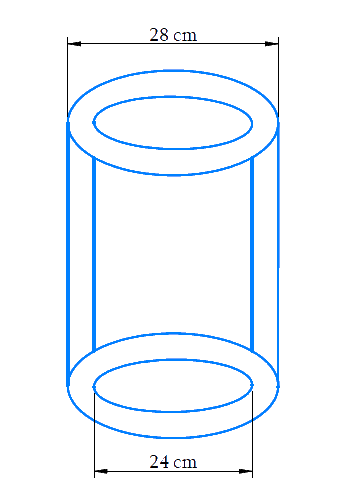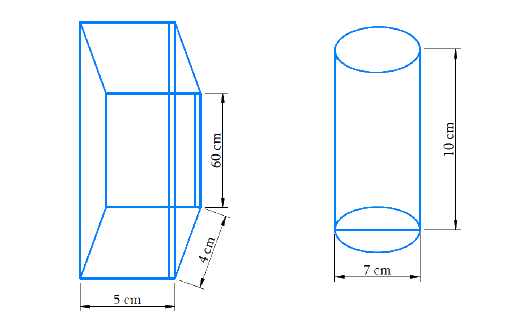# Exercise E13.6 Surface Areas and Volumes NCERT Solutions Class 9

Go back to  'Surface Areas and Volumes'

## Chapter 13 Ex.13.6 Question 1

The circumference of the base of a cylindrical vessel is $$132\; cm$$ and its height is $$25\; cm$$. How many litres of water can it hold? \begin{align}(1000\,c{m^3} = 1\,l) \end{align}

### Solution

Reasoning:

Volume of a cylinder of base radius $$r$$ and height is $$V = \pi r^{2} h$$.

What is known?

Circumference of the base and the height.

What is unknown?

Litres of water the cylindrical vessel can hold.

Steps:

Since the base of a cylindrical vessel is a circle, the circumference is $$2 \pi r=132 \mathrm{cm}$$ (given)

\begin{align} 2\pi r &= 132\\2 \times \frac{{22}}{7} \times r &= 132\\r &= \frac{{132 \times 7}}{{2 \times 22}}\\ &= 21\,\,cm \end{align}

Radius \begin{align}(r) = 21\, \rm{cm} \end{align}

Height \begin{align}(h) = 25\, \rm{cm}\end{align}

Capacity of the cylindrical vessel $$=$$ Volume of the cylindrical vessel $$=𝜋𝑟^2ℎ$$

\begin{align}&= \frac{{22}}{7} \times 21 \times 21 \times 25 \\&= 34650\,c{m^3} \end{align}

\begin{align} 1000\,c{m^3} &= 1\,\,l\\34650\,c{m^3} &= x\,\,l\\x &= \frac{{34650}}{{1000}}\\& = 34.65\,l \end{align}

Capacity of the cylindrical vessel\begin{align}= 34.65\,l \end{align}

## Chapter 13 Ex.13.6 Question 2

The inner diameter of a cylindrical wooden pipe is $$24 \;cm$$ and its outer diameter is $$28 \;cm$$. The length of the pipe is $$35\ cm$$. Find the mass of the pipe, if \begin{align}1\,\,c{m^3} \end{align} of wood has a mass of $$0.6\; g$$.

### Solution

Reasoning:

Since the cylindrical wooden pipe is made up of two concentric circles at the top and bottom, we have to find the volume of both the cylinders.

DiagramWhat is known?

Diameter of the inner and outer cylinder. Length of the cylinder.

What is unknown?

Mass of the pipe if  $$1 \mathrm{cm}^{3}$$ of pipe has mass $$6\; g$$.

Steps:

Volume of the outer cylinder $$V_{1}=\pi R^{2} h$$

Outer diameter $$(2R) = 28\; cm$$

Outer radius \begin{align}(R)=\frac{28}{2}=14 \mathrm{cm}\end{align}

Height $$(h) = 35\ cm$$

Volume of outer cylinder

\begin{align}V_{1}&=\frac{22}{7} \times 14 \times 14 \times 35\\&=21560 \;\rm{cm}^{3}\end{align}

Volume of inner cylinder $$V_{2}=\pi r^{2} h$$

Inner diameter \begin{align} \left( {2r} \right) = 24\,cm. \end{align}

Inner radius \begin{align} (r) = \frac{{24}}{2} = 12\,cm \end{align}

Height $$(h) = 35\ cm$$

Volume of outer cylinder

\begin{align} {V_2} &= \frac{{22}}{7} \times 12 \times 12 \times 35 \\&= 15840\,c{m^3} \end{align}

Volume of the wood used $$=$$ Outer volume $$-$$ Inner volume

\begin{align} &= 21560 - 15840 \\ & = 5720\;{\rm{cm}}^{\rm{3}} \\ \end{align}

Mass of  $$\mathrm{1cm}^{3} \text { pipe }=0.6 \mathrm{g}$$

Therefore,

Mass of $$5720 \,{cm}^{3}$$ pipe

\begin{align} &={ 0}{\rm{.6 \times 5720}}\\ &= 3432\,\,g\\&\quad\rm OR\\ &= 3.432\,\,kg \end{align}

Answer: Mass of the pipe $$= 3.432\ kg$$.

## Chapter 13 Ex.13.6 Question 3

A soft drink is available in two packs – (i) a tin can with a rectangular base of length $$5 \;cm$$ and width $$4\; cm$$, having a height of $$15\ cm$$ and (ii) a plastic cylinder with circular base of diameter $$7\ cm$$ and height $$10\ cm$$. Which container has greater capacity and by how much?

### Solution

Reasoning:

Volume of the cuboid is $$lbh$$ and volume of cylinder in\begin{align}\,\pi {r^2}h \end{align}

What is known?

Measurements of cuboid can. Measurements of cylindrical can.

What is unknown?

Which can is big and how much ?

Steps:

DiagramTin Can:

$$\text{Length} \; (l) = 5\ cm$$

$$\text{Breadth}\; (b) = 4\ cm$$

$$\text{Height}\; (h) = 15\ cm$$

$$\text{Capacity = Volume} = l b h$$.

\begin{align}&= 5 \times 4 \times 15 \\&= 300\,\,c{m^3} \\ \end{align}

Plastic Cylinder:

$$\text{Diameter} (2r) =7\; cm$$

Radius\begin{align} \,(r) = \frac{7}{2} \end{align}cm

$$\text{Height}\; (h) =10\; cm$$

\begin{align}\text{Capacity} &= \rm{Volume} = \pi {r^2}h\ \\ &= \frac{{22}}{7} \times \frac{7}{2} \times \frac{7}{2} \times 10\\ &= 385\,\,\,c{m^3} \end{align}

Clearly, the plastic cylinder has greater capacity than the tin container.

Difference\begin{align} = \,385 - 300 = 85\,c{m^3} \end{align}

The plastic cylindrical can have more capacity than the Tin Can by\begin{align}\, = 85\,\,c{m^3} \end{align}.

## Chapter 13 Ex.13.6 Question 4

If the lateral surface of a cylinder is  $$92.4\,\,\rm cm^2$$ and its height is $$5\; \rm cm$$, then find

(ii) its volume. (Use $$\pi = 3.14$$)

### Solution

Reasoning:

Lateral surface area of a cylinder is $$2\pi rh$$.

Volume of cylinder is $$\pi {r^2}h.$$

What is known?

Lateral surface area of the cylinder and its height.

What is unknown?

Steps:

Lateral surface area of a cylinder having $$r$$ as radius and height $$h$$ is $$2\pi rh$$

Lateral surface area  $$= 94.2\, \rm cm^2$$

$$\text{Height}\; h = 5\; \rm cm$$

\begin{align}2\pi rh &= 94.2\\2 \times 3.14 \times r \times 5 &= 94.2\\r &= \frac{{94.2}}{{5 \times 3.14 \times 2}} \\ & = 3\,\,\rm cm \end{align}

What is unknown?

Volume of the cylinder.

Steps:

$$\text{Radius}\; r = 3\; \rm cm$$

$$\text{Height}\; h = 5\; \rm cm$$

Volume of cylinder

\begin{align} & =\pi r^{2} h \\ & =3.14 \times 3 \times 3 \times 5 \\ & =141.3 \, \mathrm{cm}^{3}\end{align}

$$\text{Radius of its base} = 3\; \rm cm$$

Its volume $$= 141.3\,\, \rm cm^3$$

## Chapter 13 Ex.13.6 Question 5

It costs $$\rm Rs\; 2200$$ to paint the inner curved surface of a cylindrical vessel $$10\; \rm m$$ deep. If the cost of painting is at the rate of $$\rm Rs\; 20$$ per $$\rm m^2$$, find.

(i) inner curved surface area of the vessel,

(iii) capacity of the vessel.

### Solution

Reasoning:

The ratio between the total cost and the rate per $$\rm m^2$$ will give the inner curve surface area. Curve surface area of a cylinder is $$2\pi rh$$ if the height of the cylinder is $$h$$ and base radius is $$r$$.

What is known?

Total cost to paint and cost per $$\rm {m^2}$$. And height of the cylindrical vessel.

What is unknown?

Inner curved surface area.

Steps:

Total cost to paint $$=2200$$

Cost of paint per $$\rm {m^2}$$ $$=20$$

$$\therefore$$ Surface area \begin{align} = \frac{{2200}}{{200}} \end{align} \begin{align} = 110\,\, \rm cm^2 \end{align}

What is unknown?

Steps:

Inner curved surface =\begin{align}110\,\, \rm cm^2 \end{align}

$$\text{Height} \;(h) = 10\; \rm m$$

\begin{align}2\pi rh &= 110 \\ 2 \times \frac{{22}}{7} \times r \times 10 &= 110 \\r &= \frac{{110 \times 7}}{{22 \times 2 \times 10}}\\ &= \frac{7}{4}\\ &= 1.75\,\,\, \rm cm \end{align}

What is unknown?

Capacity of the vessel.

Steps:

Capacity of the vessel = Volume of the vessel = \begin{align}\,\pi {r^2}h \end{align}

Radius (r)\begin{align}\, = 1.75\,\,\,\rm cm \end{align}

Height (h)\begin{align}\, = 10\,\, \rm cm \end{align}

Capacity

= $$\,2 \times \frac{{22}}{7} \times 1.75 \times 1.75 \times 10$$

\begin{align} = 96.25\,\, \rm cm^3 \end{align}

The capacity of the vessel is \begin{align}\, = 96.25\,\, \rm cm^3 \end{align}

Inner curved surface area\begin{align}\,110\,\, \rm cm^2 \end{align}

Radius of the box $$=1.75\; \rm m$$

Capacity of the vessel \begin{align}\, = 96.25\,\, \rm cm^3 \end{align}

## Chapter 13 Ex.13.6 Question 6

The capacity of a closed cylindrical vessel of height $$1 \; \rm m$$ is $$15.4$$ litres. How many square metres of metal sheet would be needed to make it?

### Solution

Reasoning:

Surface area total of a closed cylinder is $$2\pi rh + 2\pi {r^2}$$.

$$2\pi rh$$ corresponds to the Curved Surface Area of the cylinder and  $$2\pi {r^2}$$ corresponds to the area of the circular lids of the cylindrical vessel in order to make it a closed container. Volume of cylinder $$\pi {r^2}h$$.

What is known?

Capacity and height of the cylinder.

What is unknown?

Metal sheet needed to make it.

Steps:

\begin{align}\text{Capacity} &= \rm{15.4 \, liters}\\ V &= \frac{{15.4}}{{1000}} = .0154\,\,\rm {m^3} \end{align}

\begin{align}\rm{Volume} &= \pi {r^2}h\\ 0.0154 &= \frac{{22}}{7} \times {r^2} \times 1 \\{r^2} &= \frac{{.0154 \times 7}}{{22}}\\{r^2} &= .0049\\r &= \sqrt {.0049} \\ &= 0.07\,\, \rm m \end{align}

Curved surface area

\begin{align} & = 2\pi rh + 2\pi {r^2} \\ & = 2\pi r(r + h) \\ & = 2 \times \frac{{22}}{7} \times 0.07 \times (0.07 + 1) \\ & = 0.4708\,\, \rm {m^2} \end{align}

$$0.47\, \rm {m^2}$$ of metal sheet would be needed.

## Chapter 13 Ex.13.6 Question 7

A lead pencil consists of a cylinder of wood with a solid cylinder of graphite filled in the interior. The diameter of the pencil is $$7 \; \rm mm$$ and the diameter of the graphite is $$1\; \rm mm$$. If the length of the pencil is $$14 \; \rm cm$$, find the volume of the wood and that of the graphite.

### Solution

Reasoning:

Volume of cylinder $$\pi {r^2}h$$

What is known?

Diameter of the pencil, diameter of graphite and length of the pencil.

What is unknown?

Volume of the wood and that if graphite.

Steps:

For cylinder graphite.

Diameter $$(2r) = 1 \; \rm mm$$

Radius \begin{align}(r) = \frac{1}{2}\,\, \rm mm \end{align}

Length of the pencil $$(h) = 14 \; \rm cm = 140 \;\rm mm$$

\begin{align}\text{Capacity}&= \text{Volume} \\ &=\,\pi {r^2}h\\ &= \frac{{22}}{7} \times \frac{1}{2} \times \frac{1}{2} \times 140\\ &= 110\,\, \rm mm^3 \end{align}

\begin{align} \rm{In} \,\,c{m^3} &= \frac{{110}}{{10 \times 10 \times 10}} = 0.11\,\, \rm cm^3 \end{align}

For cylinder of wood:

To find the volume of the wood:

Total volume of the pencil $$-$$ Volume of graphite

\begin{align}\pi {R^2}h - \pi {r^2}h \pi h({R^2} - {r^2})\end{align}

Diameter of pencil $$(2R) = 7 \; \rm mm$$

Radius $$(r) = \frac{7}{2} \rm mm$$

Length of the pencil $$(h) = 14 \; \rm cm = 140 \; \rm mm$$

Volume of wood \begin{align}=\,\pi h({R^2} - {r^2}) \end{align}

\begin{align} &= \frac{{22}}{7} \times 140 \times [{(\frac{7}{2})^2} - {(\frac{1}{2})^2}]\\ &= \frac{{22}}{7} \times 140 \times [\frac{{49}}{4} - \frac{1}{2}]\\ &= 5280\,\, \rm mm^3\\ &= \frac{{5280}}{{10 \times 10 \times 10}}\, \rm cm^3 \\ &= 5.28\,\, \rm cm^3 \end{align}

Volume of the wood $$= 5.28\,\, \rm cm^3$$

Volume of graphite $$= 0.11\,\,\, \rm cm^3$$

## Chapter 13 Ex.13.6 Question 8

A patient in a hospital is given soup daily in a cylindrical bowl of diameter $$7 \; \rm cm$$. If the bowl is filled with soup to a height of $$4 \; \rm cm$$, how much soup the hospital has to prepare daily to serve $$250$$ patients?

### Solution

Reasoning:

Volume of cylinder \begin{align} = \pi {r^2}h \end{align}. So, the amount of soup to be prepared will be the product of volume of the bowl (with $$h = 4\; \rm cm$$) and total number of patients.

What is known?

Diameter of the bowl and length of the soup, number of patients.

What is unknown?

Total soup to be prepared.

Steps:

Volume of soup bowl $$= \pi {r^2}h$$

Diameter $$(2R) = 7 \; \rm cm$$

Radius $$(r) = \frac{7}{2}\,\,\, \rm cm$$

Height $$(h) = 4\; \rm cm$$

$$\therefore$$ Volume of soup in one bowl

\begin{align} &= \pi {r^2}h \\ &= \frac{{22}}{7} \times \frac{7}{2} \times \frac{7}{2} \times 4\\ &= 154\,\, \rm cm^3 \end{align}

Volume of soup for $$1$$ patient $$= 154\,\, \rm cm^3$$

Volume of soup for $$250$$ patients

\begin{align} &= 154 \times 250\\ & = 38500\,\, \rm cm^3 \end{align}

Volume of soup to be prepared for $$250$$ patients is
\begin{align} = 38500\,\, \rm cm^3 \end{align} or $$38.5$$ litres [as $$1000$$ $$\rm cm^3$$ $$= 1$$ litre]